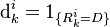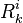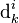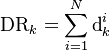# Default Rate Process

## Definition

Default Rate Process is any stochastic process that aims to model the arrival of default events in a Credit Portfolio

A default rate process is related to the corresponding Credit Loss Process which incorporates additional Loss Given Default considerations

## Formula

A default rate process is modelled as the cumulation of individual transitions of Credit Rating into the defaulted state. The individual default indicator for an entity$i$ is simply$\mbox{d}_k^i = 1_{\{ R_k^i = D\}}$

where$R_k^i$ is the rating state at observation point k

The default rate process is the sum (defined on a fixed cohort basis) of individual random variables$\mbox{d}_k^i$$\mbox{DR}_k = \sum_{i=1}^{N} \mbox{d}_k^i$